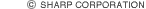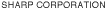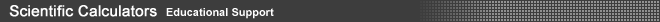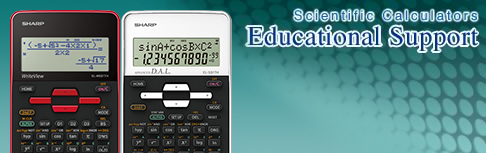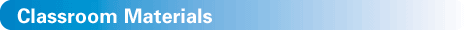Handbook vol.1 (Algebra)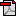Handbook vol.1 (Algebra) for EL-9650/9600c/9450/9400 (1.4M)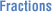Fractions and Decimals (20K)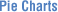Pie Charts and Proportions (20K)Shifting a Graph of Quadratic Equations (48K)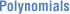Graphing Polynomials and Tracing to Find the Roots (56K)Graphing Polynomials and Jumping to Find the Roots (56K)Solving Double Inequalities (44K)System of Two-Variable Inequalities (40K)Graphing Solution Region of Inequalities (56K)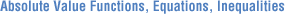Shifting a Graph of Absolute Value Functions (60K)Solving Absolute Value Equations (32K)Solving Absolute Value Inequalities (60K)Evaluating Absolute Value Functions (44K)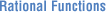Graphing Rational Functions (52K)Solving Rational Function Inequalities (40K)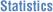Statistics calculations (132K)Rectangular coordinate graphs (68K)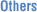CALC function (84K)

 Handbook vol.2 (Programmes)Handbook vol.2 (Programmes) for EL-9650/9600c/9450/9400 (692K)